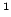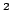Statistica Sinica 20 (2010), 927-1010

ENVELOPE MODELS FOR PARSIMONIOUS AND EFFICIENT

MULTIVARIATE LINEAR REGRESSION

R. Dennis Cook, Bing Liand Francesca ChiaromonteUniversity of Minnesota andPennsylvania State University

Abstract: We propose a new parsimonious version of the classical multivariate normal linear model, yielding a maximum likelihood estimator (MLE) that is asymptotically less variable than the MLE based on the usual model. Our approach is based on the construction of a link between the mean function and the covariance matrix, using the minimal reducing subspace of the latter that accommodates the former. This leads to a multivariate regression model that we call the envelope model, where the number of parameters is maximally reduced. The MLE from the envelope model can be substantially less variable than the usual MLE, especially when the mean function varies in directions that are orthogonal to the directions of maximum variation for the covariance matrix.

Key words and phrases: Discriminant analysis, functional data analysis, grassmann manifolds, invariant subspaces, principal components, reduced rank regression, reducing subspaces, sufficient dimension reduction.